Courses

## 20 Questions MCQ Test Mathematics (Maths) Class 8 | Olympiad Test: Understanding Quadrilaterals

Description
This mock test of Olympiad Test: Understanding Quadrilaterals for Class 8 helps you for every Class 8 entrance exam. This contains 20 Multiple Choice Questions for Class 8 Olympiad Test: Understanding Quadrilaterals (mcq) to study with solutions a complete question bank. The solved questions answers in this Olympiad Test: Understanding Quadrilaterals quiz give you a good mix of easy questions and tough questions. Class 8 students definitely take this Olympiad Test: Understanding Quadrilaterals exercise for a better result in the exam. You can find other Olympiad Test: Understanding Quadrilaterals extra questions, long questions & short questions for Class 8 on EduRev as well by searching above.
QUESTION: 1

Solution:
QUESTION: 2

Solution:
QUESTION: 3

### The diagonal of a rectangle is 10 cm and its breadth is6 cm. What is its length?

Solution:

Apply Pythagoras Theorem,
100 = 36+(Length)2
Length=8cm

QUESTION: 4

ABCD and MNOP are quadrilaterals as shown in the figure.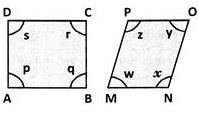Which of the following is correct?

Solution:
QUESTION: 5

What do you call a parallelogram which has equal diagonals?

Solution:
QUESTION: 6

In a square ABCD, the diagonals bisect at O. What type of a triangle is AOB?

Solution:

Since diagonals of a square are equal and bisect at right angles, triangle AOB is an isosceles right angled triangle.

QUESTION: 7

The perimeter of a parallelogram is180 cm. If one side exceeds the other by 10 cm, what are the sides of the parallelogram?

Solution:

Let one side of the parallelogram be 'x' cm. Then adjacent side is (x+10)cm.

∴ Perimeter = x+(x+10)+x+(x+10) = 180

(Given) ⇒ 4x+20 = 180 or x = 40cm

∴ x+10 = 50cm

QUESTION: 8

In the quadrilateral ABCD, the diagonals AC and BD are equal and perpendicular to each other. What type of a quadrilateral is ABCD?

Solution:

A quadrilateral in which the diagonals are equal and perpendicular is called a square.

QUESTION: 9

ABCD is a parallelogram as shown in the figure. If AB = 2AD and P is the mid-point of AB, what is the measure of ∠CPD ?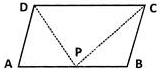Solution:

As shown in the figure, since P is the midpoint of AB and AB = 2AD,we have AB = 2AP = 2AD or  AP = AD. i.e.,
triangle ADP is an isosceles triangle. If ∠ADP = xo and ∠APD = xo, then ∠A = 180o−2xo.
Since ∠B is adjacent to ∠A, in ABCD ∠B = 180o−(180o−2x) = 2x. In ΔCBP,xo+xo+2x= 180o(Angle sum property)

⇒ 4xo = 180⇒ x= 45o ∴∠CPD = 180o−2xo = 180o−2×45= 90o

QUESTION: 10

In a parallelogram ABCD, if AB = 2x+5, CD = y+1,  AD= y+5  and BC = 3x−4,what is the ratio of AB and BC?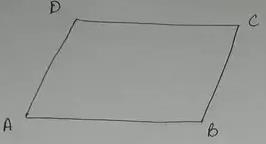Solution:

We know that in a parallelogram opposite sides are equal.

∴ AB = CD or  2x+5=y+1 and

2x−y =−4 ....(i)
y−3x = −9 .....(ii)

we get −x =−13 or x = 13

and y = 30.

Substituting, we have

AB = 31 cm and BC = 35 cm

∴The required ratio = 31:35

QUESTION: 11

If ABCD is an isosceles trapezium, what is the measure of ∠C?

Solution: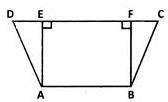From definition, we know that in an isosceles trapezium the non-parallel sides are equal or AD = BC in the  figure.  Drop perpendiculars AE and BF to CD. Triangles AED and BFC are congruent by R.H.S congruency. Hence, ∠D = ∠C

QUESTION: 12

A diagonal of a rectangle is inclined to one side of the rectangle at 25o. What is the measure of the acute angle between the diagonals?

Solution: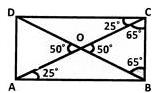Since ∠CAB = 25o ∠CAB = 65o

Let diagonals meet at O. ΔOCB is an isosceles triangle.

∴ ∠OBC = 65o

⇒ ∠BOC = 50o

QUESTION: 13

If angles P, Q, R and S of the quadrilateral PQRS, taken in order, are in the ratio 3:7:6:4, what is PQRS?

Solution:

Let the angles be 3x, 7x , 6x and 4x.
∴ 3x+7x+6x+4x = 360o or 20x = 360o or x = 18o.
The angles are 54o,126o,108o and 72o.
We see that adjacent angles are supplementary but opposite angles-are not equal. Clearly, it is a trapezium.

QUESTION: 14

If AB and CD are diameters, what is ACBD?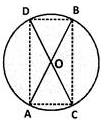Solution:

Since the angle in a semicircle is a right angle, clearly ∠A = ∠C = ∠B = ∠D = 90o
The diagonals (diameters) are equal but they are not intersecting (bisecting) at right angles. Hence, it is not a square and can be only a rectangle.

QUESTION: 15

If two adjacent angles of a parallelogram are in the ratio 3:2, what are their measures?

Solution:

Let the angles be 3x and 2x. We have, 3x+2x = 180o ⇒ 5x = 180o ⇒ x = 36o
∴ The angles are 36o×3 and 36o×2 = 108o and 72o.

QUESTION: 16

ABC and DEF are straight lines.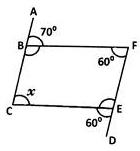Find the value of 'x',

Solution:

In the given figure. ∠ABF+∠FBC = 180o

70o+∠FBC = 180o ⇒ ∠FBC = 180o−70= 110o

Now,  ∠DEC+∠CEF = 180o

∠CEF = 180o−60= 120o

Now, ∠FBC+∠BCE+∠CEF+∠BFE = 36o

290o+x = 360⇒ x = 70o

QUESTION: 17

ABCD is a rectangle. Its diagonals meet at O.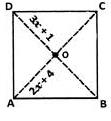Find x; if OA = 2x+4 and OD = 3x+1.

Solution:

OD is half of the diagonal BD and OA  is half of the diagonal AC. Diagonals are equal. So, their halves are also equal. Therefore, 3x + 1 = 2x + 4
⇒ x = 3.

QUESTION: 18

ABCD is a rhombus.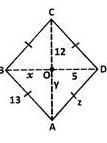Find the respective values of x, y and z.

Solution:

x = OB = OD (Diagonals bisect) = 5
y = OA = OC (Diagonals bisect) = 12
z = side of the rhombus = 13 (All sides are equal).

QUESTION: 19

In the figure, ABCD is a parallelogram.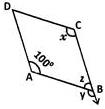Find the respective values of x, y and z.

Solution:

C is opposite to A. So,
x = 100o (Opposite angles property.)
y = 100o (Measure of angle corresponding to ∠x.)
z = 80o (Since ∠y,∠z is a linear pair)

QUESTION: 20

In the figure, ABCD is a rhombus and ABDE is a parallelogram.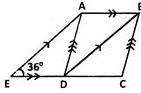Given that EDC is a straight line and ∠AED = 36o find ∠BAD.

Solution:

BDC = AED = 36o (Corresponding  s, AE  BD.) ABD= BDC = 36o (Alternate  s, AB DC) ADB = ABD = 36o (Base angles of isosceles, since AB = DC) BAD = 180o−ABD−ADB (Angle sum of a triangle.) = 180o−36o−36o = 108o